# NCERT Solutions For Class 9 Math Chapter – 9 Exercise – 9.2

NCERT Solutions For Class 9 Math Chapter – 9 Exercise – 9.2

Q1. In Figure ABCD is a parallelogram , AE bisects DC and CF bisects AD. If AB = 16 cm , AE = 8 cm and CF = 10 cm Find AD.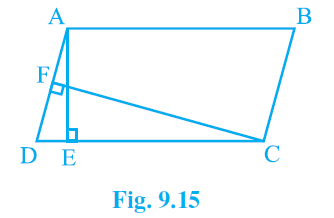Solution:-

AD X CF = AE X CD  (I)

AB = CD = 16 cm

AD X 10 = 8 X 16 = AD = 12.8 cm.

Q2. If E , F , G and H are respectively the mid – points of the sides of a parallelogram ABCD Show that Area(EFGH) = 1/2 area(ABCD).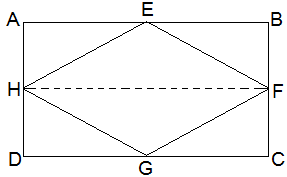Solution:-

In Parallelogram ABCD,

AB= CD and AB // CD.

1/2AB = 1/2CD

DG = AE   [E and G are mid points of AB and CD]

We have DG = AE and DG // AE.

AEGD is a parallelogram

Now parallelogram AEGD and triangle HEG are on the same base and between the same parallels.

Area (HEG) = 1/2Area(AEGD)   (I)

Similarly we can show that

Area(FGE) = 1/2Area(EBCG)    (ii)

From (I) and (ii) we get

Area(HEG) + Area(FGE) = 1/2Area (AEGD) + Area(EBCG)

Area(EFGH) = 1/2Area(ABCD)

Q3. P and Q are any two points lying on the sides DC and AD respectively of a parallelogram ABCD. Show that Area(APB) = Area(BQC).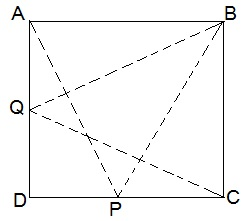Solution:-

Area(APB) = 1/2Area(ABCD) (I)

Area(QBC) = 1/2Area(ABCD) (ii)

Hence Area(APB) = Area(QBC)   [From (I) and (ii)]

Q4. In figure P is a point in the interior of a parallelogram ABCD. Show that

• Area(APB) + Area(PCD) = 1/2Area (ABCD)
• Area(APD) + Area(PBC) = Area(APB) + Area(PCD)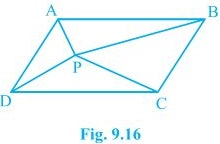Solution:-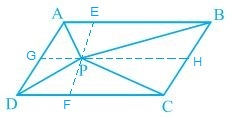AD // BC  [Opposite sides of a parallelogram]

AL // BM  (I)

AB // LM   (ii)

ABML is a parallelogram [From (I) and (ii)]

Area(APB) = 1/2Area(ABML)   (iii)

Similarly we can show that

Area(PDC) = 1/2Area(LMCD)    (iv)

Adding (iii) and (iv) we get

Area(APB) + Area(PDC) = 1/2Area(ABML) + 1/2Area(LMCD)

= 1/2[Area(ABML) + Area(LMCD)]

= Area(APB) + Area(PDC) = 1/2Area(ABCD)

( ii ) Area(APD) + Area(BPC) = 1/2Area(ABCD)

Area(APD) + Area(BPC) = Area(APB) + Area(PDC(

Q5. In figure PQRS and ABRS are parallelogram and X is any point on side BR. Show that

• Area(PQRS) = Area(ABRS)
• Area(AXS) = 1/2Area(PQRS).

Solution:-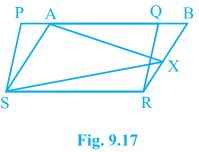• As parallelogram PQRS and ABRS are on the same base base SR and between the same parallel SR and PB.

Hence Area(PQRS) = Area(ABRS).

• Area(AXS) = 1/2Area(ABRS)

But Area(ABRS) =  Area(PQRS)

Area(AXS) = 1/2Area(PQRS).

Q6.  A  farmer was having a field in the form of a parallelogram PQRS. She took any point A on RS and joined it to points  P and Q In how many parts the fields is divided ? What are the shapes of these parts ?  The farmer wants to sow wheat and pulses in equal portions of the field separately. How  should she do it ?

Solution:-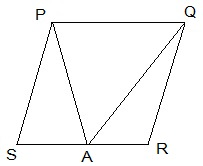Area(PAQ) = 1/2Area(PQRS)   (I)

Area(PSA) + Area(QAR) = 1/2Area(PQRS)  (ii)

From (I) and (ii) farmer has two options:

Option 1: Wheat in Area(PAQ) and pulses in Area(PSA) + Area(QAR).

Option ii: Pulses in Area(PAQ) and wheat in Area(PSA) + Area(QAR).